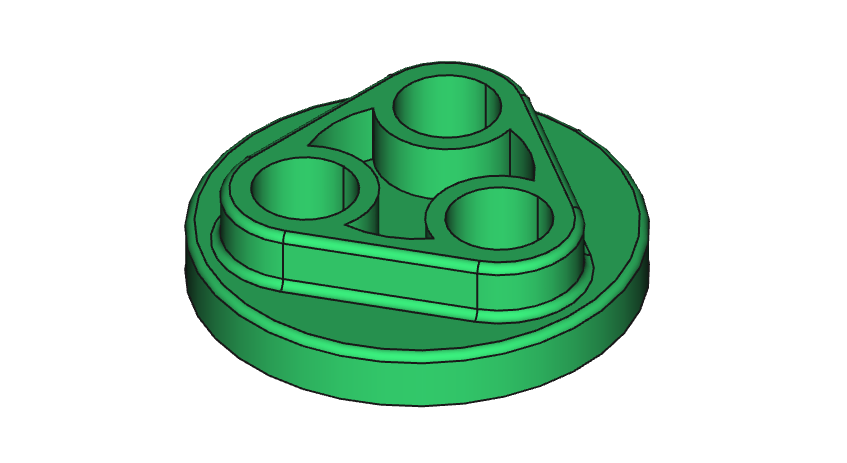# FreeCAD Basic Exercise #4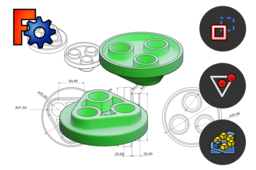This tutorial introduces the Construction Mode for constraining sketch geometry and also new features: Polygon, Equal and Fillet (in Sketcher) then Thickness and Polar Pattern (in Part Design).

1. ### Step 1: Presentation of the exercise

We will model the part illustrated below by the method proposed in the tree structure.

For the dimensions of the part you can refer to the drawing below but they will be repeated in the modelling procedure.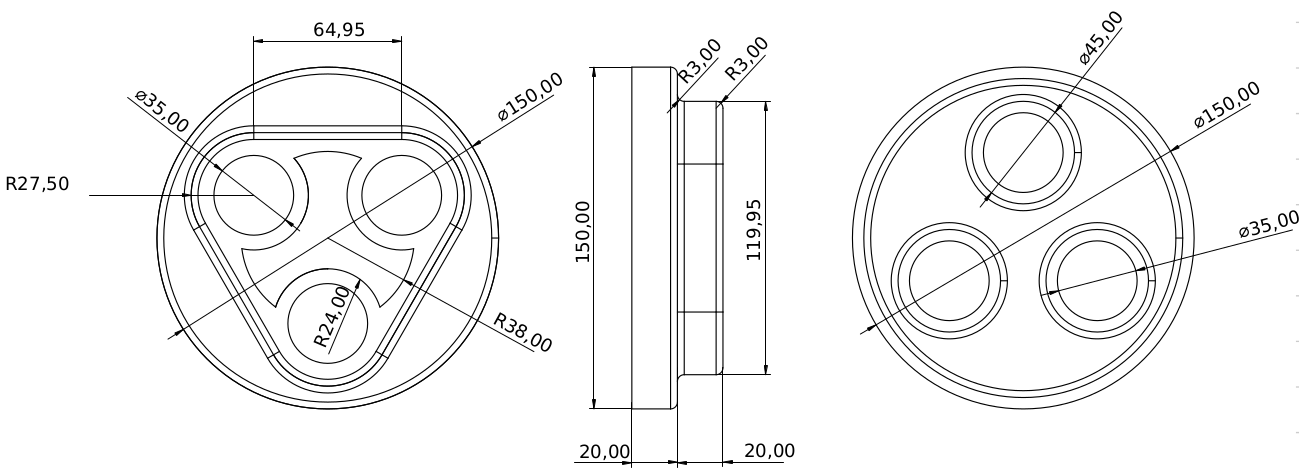2. ### Step 2: Modelling procedure

1. Create a cylinder with a diameter of 150 mm and a height of 20 mm with the functions Circle (coincident with the centre of the marker) and Pad.2. Create three circles of the same diameter, equidistant and centred around the axis of the cylinder following this steps :

A) Activate Construction Mode and select Polygon → Triangle to make a construction triangle centred on the cylinder axis.

B) Place a horizontality constraint to align the height of the triangle with the marker.

C) Turn off Construction Mode and then create three circles coincident with the vertices of the triangle.

D) Create an Equality constraint between the three circles.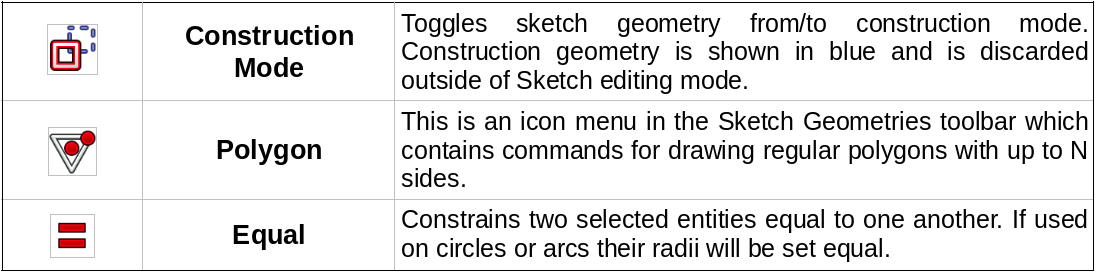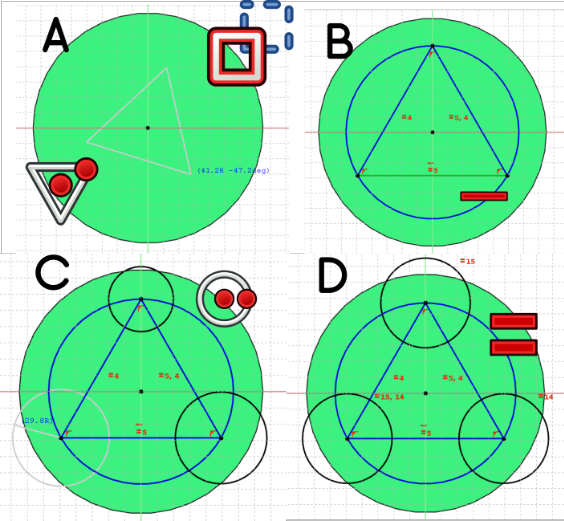3. Add diameter constraints of 35 mm on the sketch circles and 75 mm on the construction circle.

4. Apply the Pocket function "Through All".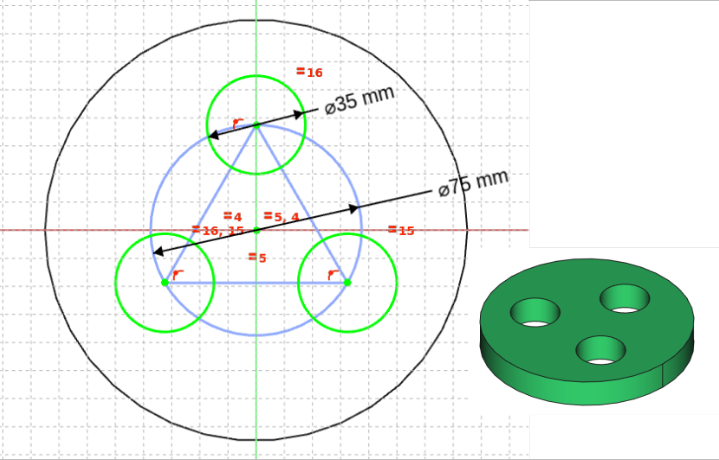5. Click on the top face and use the Thickness function with the following settings: Layer (default setting), Intersection (to keep sharp edges) and Generate thickness inwards (to shift the faces inwards).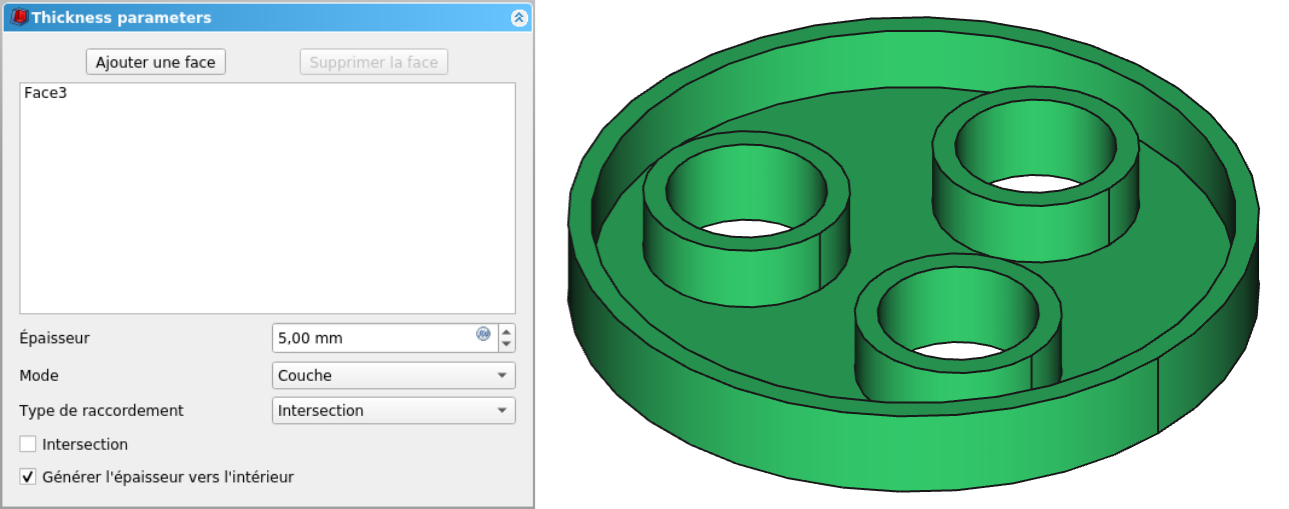6. In a new sketch, create a triangle with rounded edges using the Fillet function. The triangle will then need to be connected to the construction circle and an arc constraint created as shown below.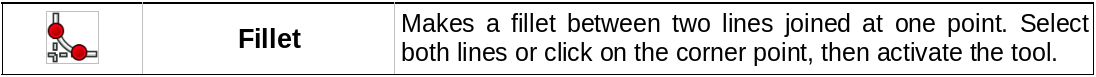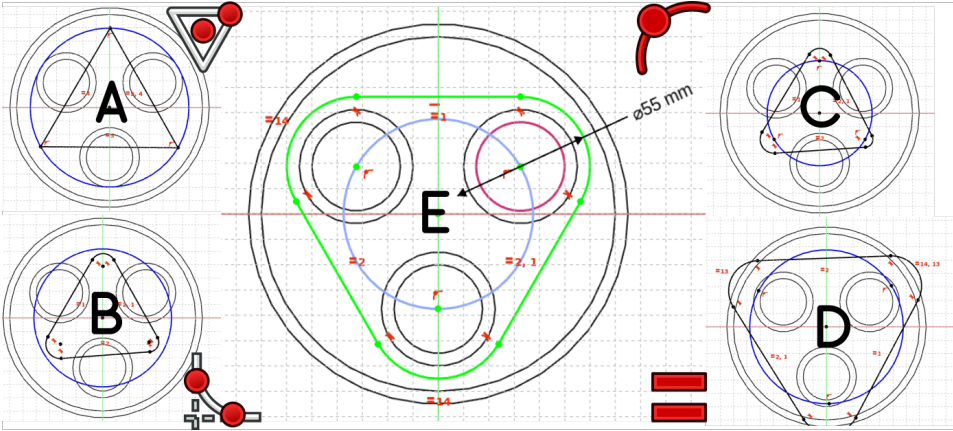7. To keep the holes open, create a Pocket by selecting the circular faces at the bottom of the holes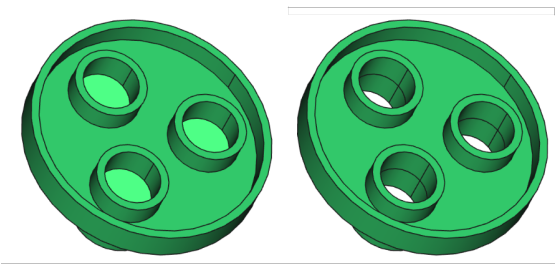8. Create the sketch below with the Arc and External Geometry functions. Apply a 5 mm pocket.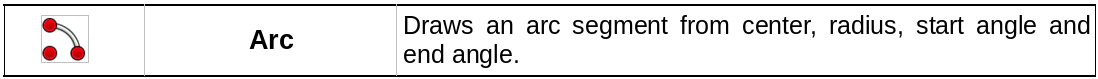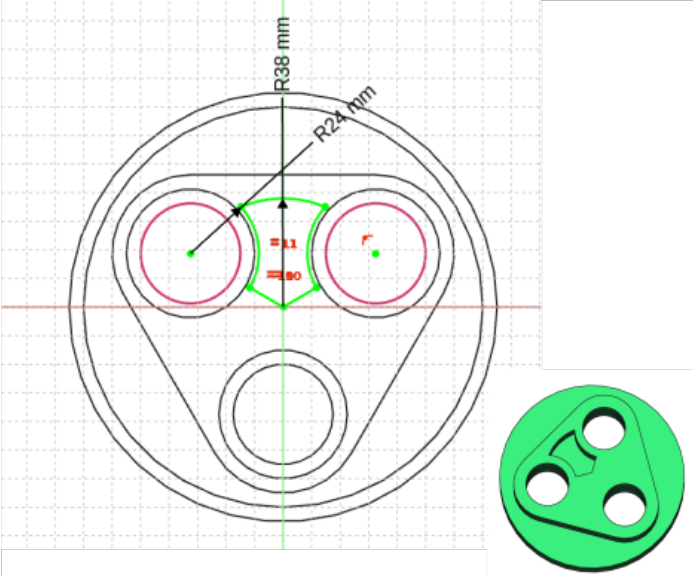9. Use the Polar Pattern function on the newly created pocket with an angle of 360° (default) and a number of occurrences of 3.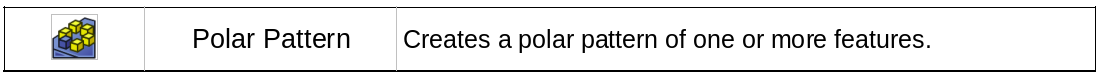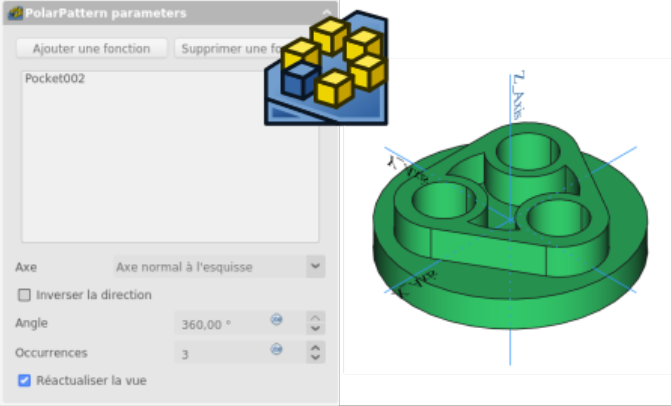9. Add edge fillets to finalise the part.